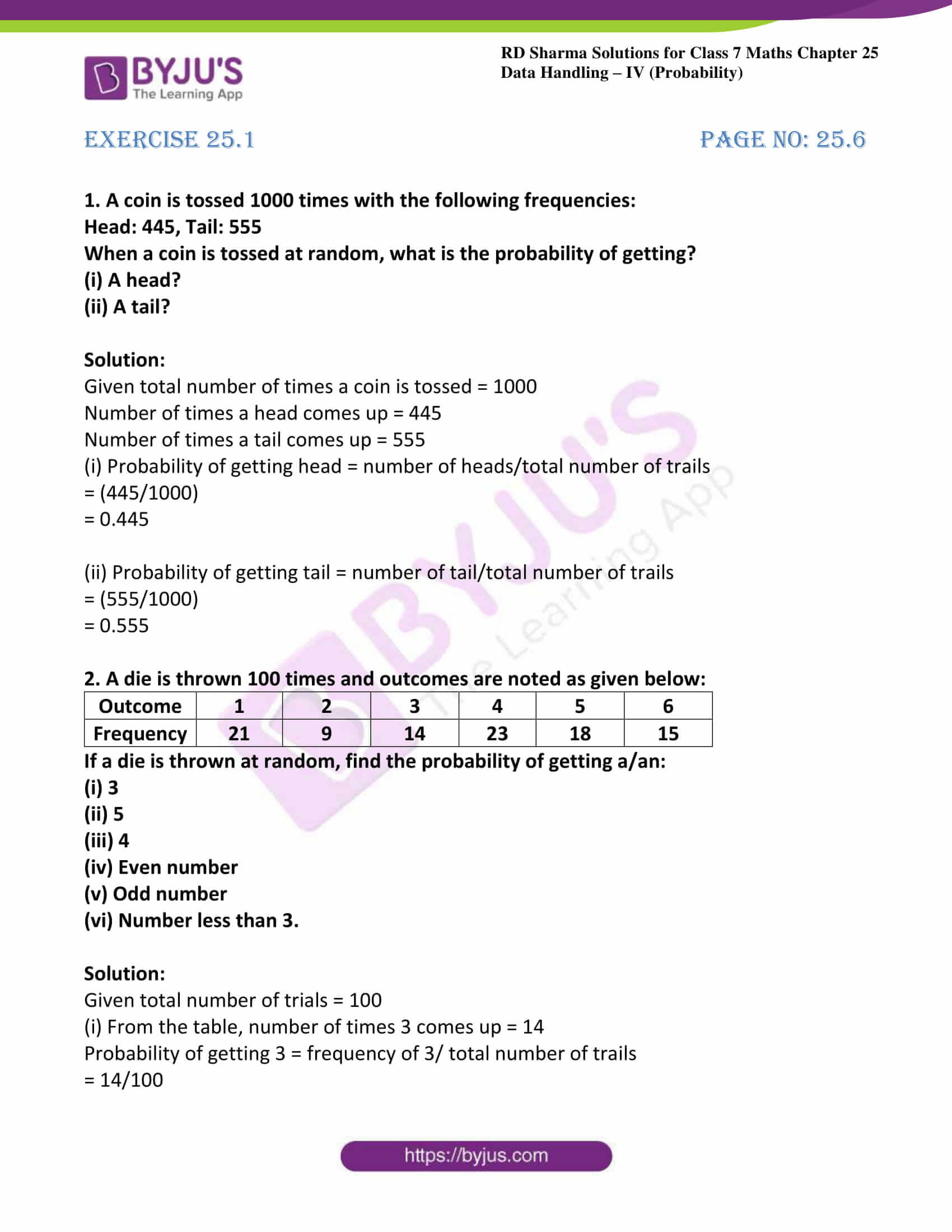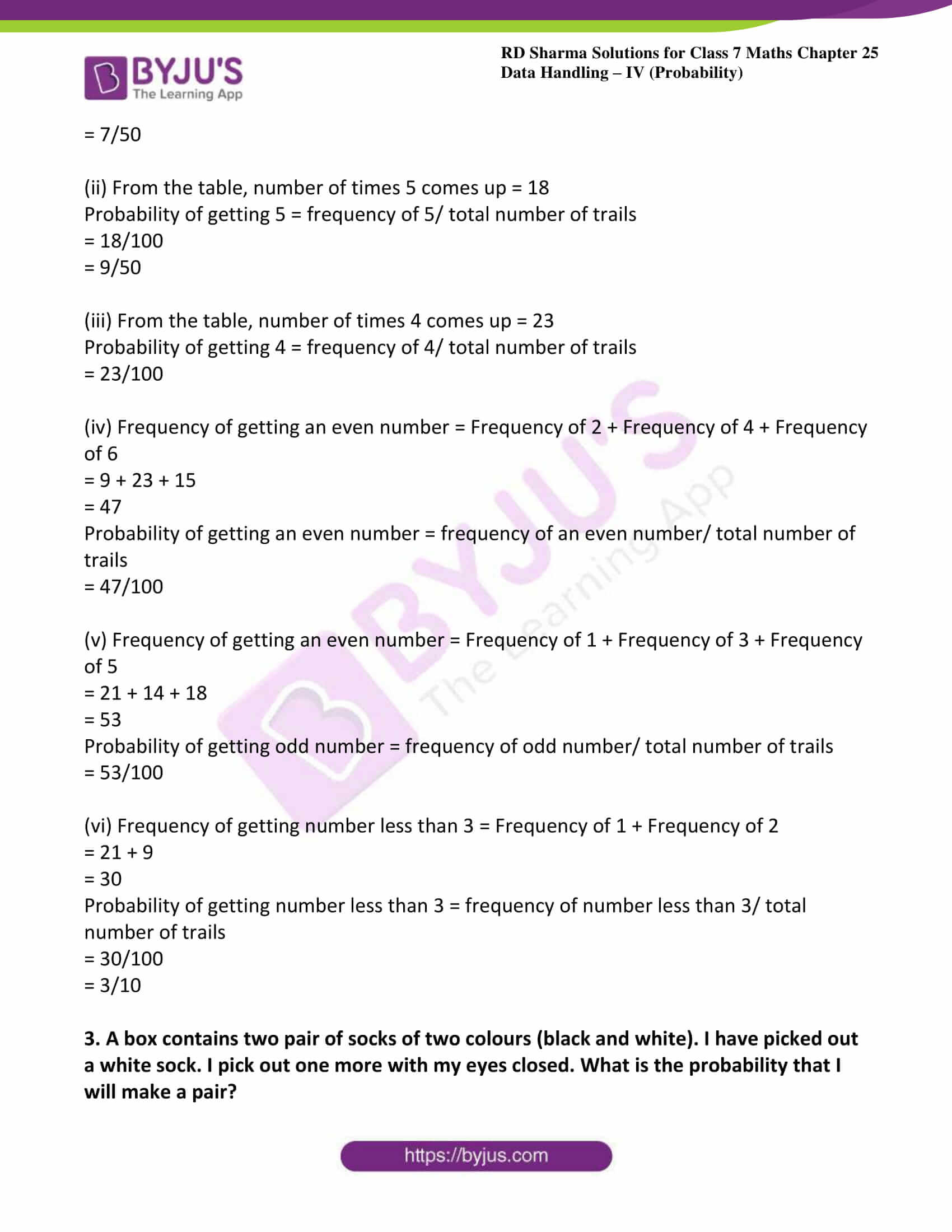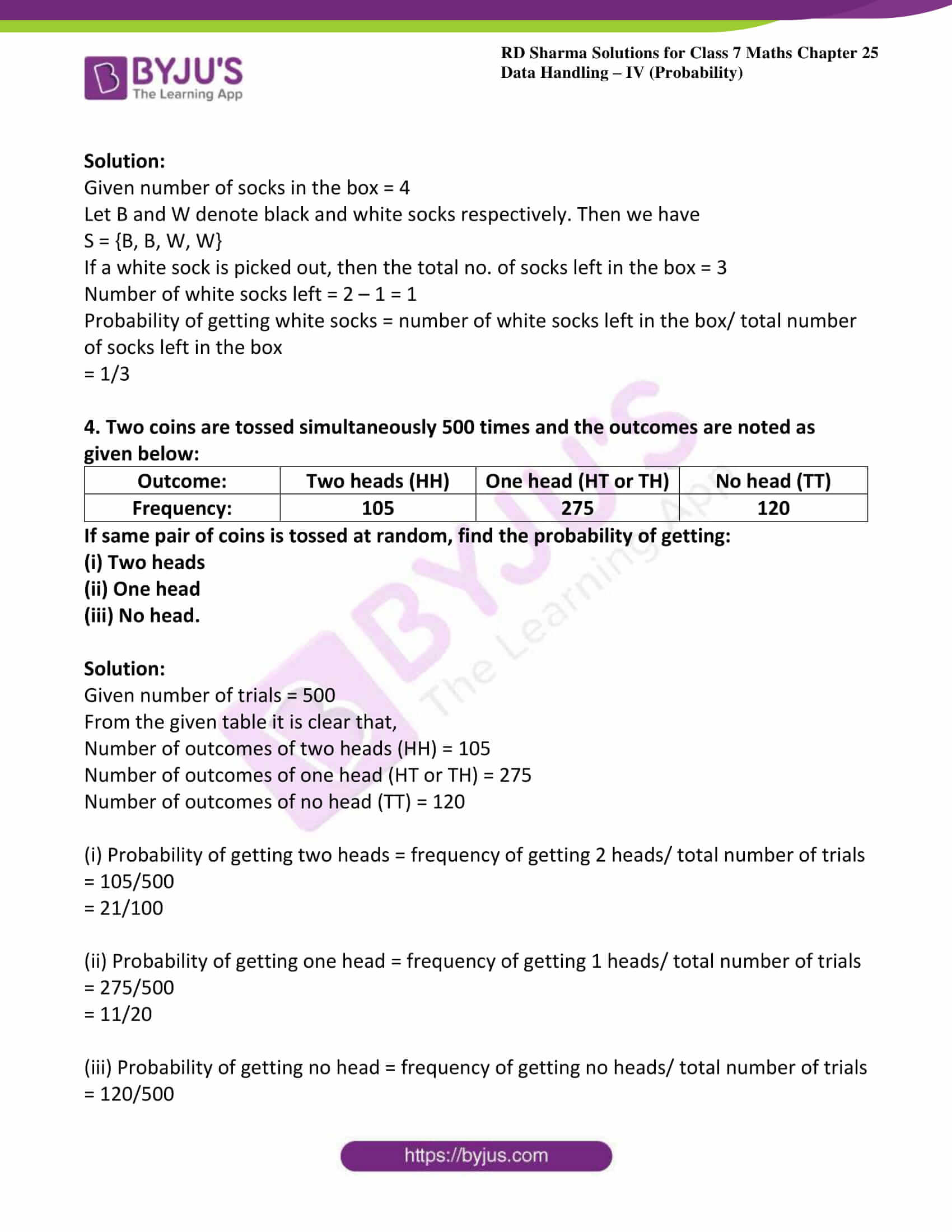# RD Sharma Solutions for Class 7 Maths Chapter 25 Data Handling - IV (Probability)

In order to excel in exams, students can view and download the PDF of RD Sharma Solutions for Class 7 Maths Chapter 25 Data Handling – IV (Probability). RD Sharma Solutions are useful for students as it helps them in scoring high marks in the examination. These solutions are prepared by subject matter experts at BYJU’S, describing the complete method of solving problems. RD Sharma Solutions for Class 7 given here contains solutions to all the problems present in this chapter. This helps students to get a good score in the examinations, while also providing extensive knowledge about the subject. Let us have a look at some important topics covered in this chapter.

• Definition and meaning of probability
• Definition of trial
• Definition of event
• Random experiment
• Happening of an event
• Empirical probability

## Download the PDF of RD Sharma Solutions For Class 7 Maths Chapter 25 Data Handling – IV (Probability)### Access answers to Maths RD Sharma Solutions For Class 7 Chapter 25 – Data Handling – IV (Probability)

1. A coin is tossed 1000 times with the following frequencies:

When a coin is tossed at random, what is the probability of getting?

(ii) A tail?

Solution:

Given total number of times a coin is tossed = 1000

Number of times a head comes up = 445

Number of times a tail comes up = 555

(i) Probability of getting head = number of heads/total number of trails

= (445/1000)

= 0.445

(ii) Probability of getting tail = number of tail/total number of trails

= (555/1000)

= 0.555

2. A die is thrown 100 times and outcomes are noted as given below:

 Outcome 1 2 3 4 5 6 Frequency 21 9 14 23 18 15

If a die is thrown at random, find the probability of getting a/an:

(i) 3

(ii) 5

(iii) 4

(iv) Even number

(v) Odd number

(vi) Number less than 3.

Solution:

Given total number of trials = 100

(i) From the table, number of times 3 comes up = 14

Probability of getting 3 = frequency of 3/ total number of trails

= 14/100

= 7/50

(ii) From the table, number of times 5 comes up = 18

Probability of getting 5 = frequency of 5/ total number of trails

= 18/100

= 9/50

(iii) From the table, number of times 4 comes up = 23

Probability of getting 4 = frequency of 4/ total number of trails

= 23/100

(iv) Frequency of getting an even number = Frequency of 2 + Frequency of 4 + Frequency of 6

= 9 + 23 + 15

= 47

Probability of getting an even number = frequency of an even number/ total number of trails

= 47/100

(v) Frequency of getting an even number = Frequency of 1 + Frequency of 3 + Frequency of 5

= 21 + 14 + 18

= 53

Probability of getting odd number = frequency of odd number/ total number of trails

= 53/100

(vi) Frequency of getting number less than 3 = Frequency of 1 + Frequency of 2

= 21 + 9

= 30

Probability of getting number less than 3 = frequency of number less than 3/ total number of trails

= 30/100

= 3/10

3. A box contains two pair of socks of two colours (black and white). I have picked out a white sock. I pick out one more with my eyes closed. What is the probability that I will make a pair?

Solution:

Given number of socks in the box = 4

Let B and W denote black and white socks respectively. Then we have

S = {B, B, W, W}

If a white sock is picked out, then the total no. of socks left in the box = 3

Number of white socks left = 2 – 1 = 1

Probability of getting white socks = number of white socks left in the box/ total number of socks left in the box

= 1/3

4. Two coins are tossed simultaneously 500 times and the outcomes are noted as given below:

If same pair of coins is tossed at random, find the probability of getting:

Solution:

Given number of trials = 500

From the given table it is clear that,

Number of outcomes of two heads (HH) = 105

Number of outcomes of one head (HT or TH) = 275

Number of outcomes of no head (TT) = 120

(i) Probability of getting two heads = frequency of getting 2 heads/ total number of trials

= 105/500

= 21/100

(ii) Probability of getting one head = frequency of getting 1 heads/ total number of trials

= 275/500

= 11/20

(iii) Probability of getting no head = frequency of getting no heads/ total number of trials

= 120/500

= 6/25